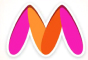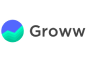New update is available. Click here to update.
Topics

# Best Time to Buy and Sell Stock III

Hard0/120+27 more companies

## Problem statement

You are Harshad Mehta’s friend. He told you the price of a particular stock for the next ‘n’ days.

You are given an array ‘prices’ which such that ‘prices[i]’ denotes the price of the stock on the ith day.

You don't want to do more than 2 transactions. Find the maximum profit that you can earn from these transactions.

Note

``````1. Buying a stock and then selling it is called one transaction.

2. You are not allowed to do multiple transactions at the same time. This means you have to sell the stock before buying it again.
``````
Example:
``````Input: ‘n’ = 7, ‘prices’ = [3, 3, 5, 0, 3, 1, 4].

Output: 6

Explanation:
The maximum profit can be earned by:
Transaction 1: Buying the stock on day 4 (price 0) and then selling it on day 5 (price 3).
Transaction 2: Buying the stock on day 6 (price 1) and then selling it on day 6 (price 4).
Total profit earned will be (3 - 0) + ( 4 - 1) = 6.
``````
Detailed explanation ( Input/output format, Notes, Images )
Sample Input 1:
``````6
1 3 1 2 4 8
``````
Sample Output 1:
``````9
``````
Explanation Of Sample Input 1 :
``````The maximum profit can be earned by:
Transaction 1: Buying the stock on day 1 (price 1) and then selling it on day 2 (price 3).
Transaction 2: Buying the stock on day 3 (price 1) and then selling it on day 6 (price 8).
Total profit earned will be (3 - 1) + ( 8 - 1) = 9.
``````
Sample Input 2:
``````5
5 4 3 2 1
``````
Sample Output 2:
``````0
``````
Explanation of Sample Output 2:
``````It's better not to do any transactions as the stock prices are decreasing.
``````
Expected time complexity:
``````The expected time complexity is O(n).
``````
Constraints:
``````1 <= ‘n’ <= 10^6
0 <= ‘prices[i]’ <= 10^3

Where ‘prices[i]’ is the stock price on ith day.

Time Limit: 1 sec.
``````Console